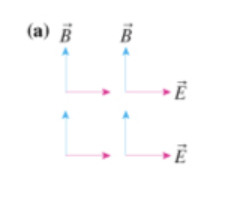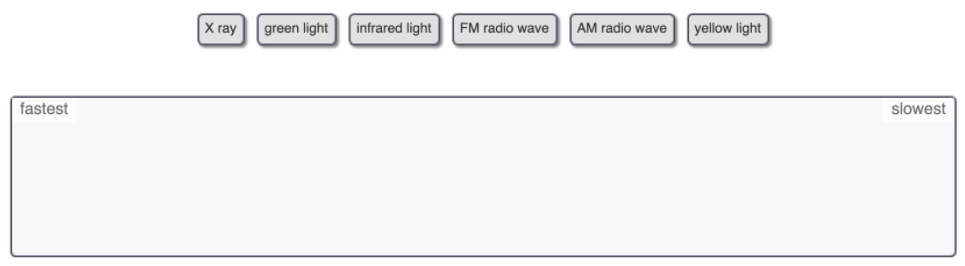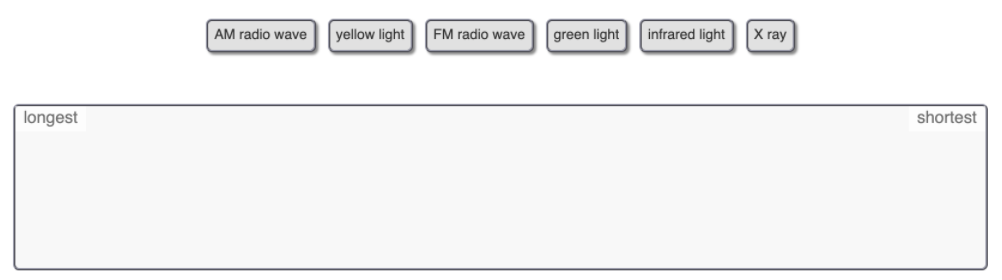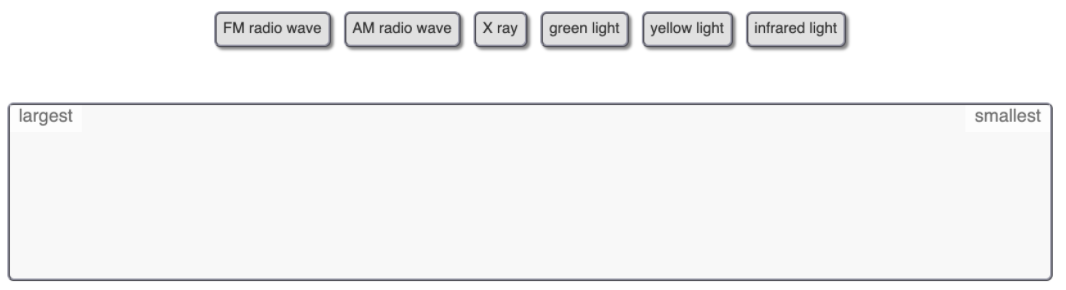# Problem: Part A. In what direction is the electromagnetic wave traveling in the figure (Figure 1)a?Part B. In what direction is the electromagnetic wave traveling in the figure (Figure 2)b?Part C. Rank these electromagnetic waves on the basis of their speed (in vacuum). Rank from fastest to slowest. To rank items ad equivalent, overlap them.Part D. Rank these electromagnetic waves on the basis of their wavelength. Rank from longest to shortest. To rank items ad equivalent, overlap them.Part E. Rank these electromagnetic waves on the basis of their frequency. Rank from largest to smallest. To rank items ad equivalent, overlap them.

###### FREE Expert Solution

In this problem, we'll consider the equation:

$\overline{){\mathbf{S}}{\mathbf{=}}\frac{\mathbf{1}}{{\mathbf{\mu }}_{\mathbf{0}}}{\mathbf{E}}{\mathbf{×}}{\mathbf{B}}}$, where S is the vector describing the rate of energy transport per unit area, E is the electric field, and B is the magnetic field.

Part A

Using the equation:

$\begin{array}{rcl}\mathbf{S}& \mathbf{=}& \frac{\mathbf{1}}{{\mathbf{\mu }}_{\mathbf{0}}}\mathbf{E}\mathbf{×}\mathbf{B}\\ & \mathbf{=}& \mathbf{i}\mathbf{×}\mathbf{j}\\ & \mathbf{=}& \mathbf{k}\end{array}$

89% (144 ratings)###### Problem Details

Part A. In what direction is the electromagnetic wave traveling in the figure (Figure 1)a?Part B. In what direction is the electromagnetic wave traveling in the figure (Figure 2)b?Part C. Rank these electromagnetic waves on the basis of their speed (in vacuum). Rank from fastest to slowest. To rank items ad equivalent, overlap them.Part D. Rank these electromagnetic waves on the basis of their wavelength. Rank from longest to shortest. To rank items ad equivalent, overlap them.Part E. Rank these electromagnetic waves on the basis of their frequency. Rank from largest to smallest. To rank items ad equivalent, overlap them.